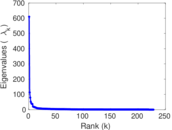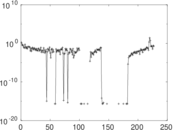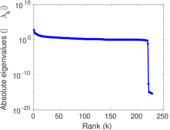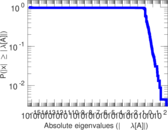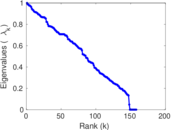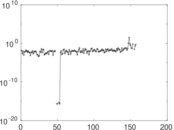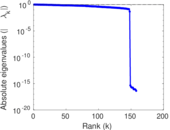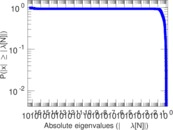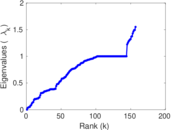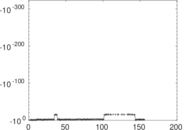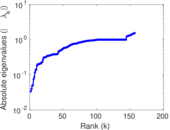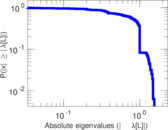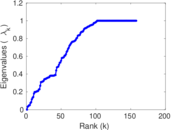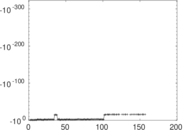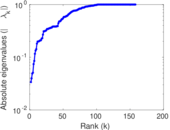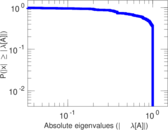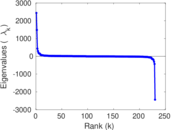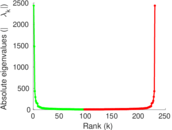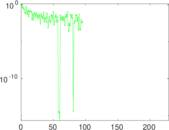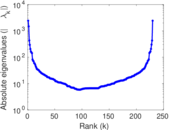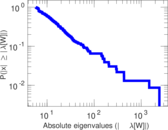# Wikiquote edits (is)

This is the bipartite edit network of the Icelandic Wikiquote. It contains users and pages from the Icelandic Wikiquote, connected by edit events. Each edge represents an edit. The dataset includes the timestamp of each edit.

 Code `qis` Internal name `edit-iswikiquote` Name Wikiquote edits (is) Data source http://dumps.wikimedia.org/ AvailabilityDataset is available for download Consistency checkDataset passed all tests Category Authorship network Dataset timestamp 2017-10-20 Node meaning User, article Edge meaning Edit Network formatBipartite, undirected Edge typeUnweighted, multiple edges Temporal dataEdges are annotated with timestamps

## Statistics

 Size n = 1,490 Left size n1 = 230 Right size n2 = 1,260 Volume m = 5,743 Unique edge count m̿ = 2,791 Wedge count s = 269,410 Claw count z = 37,287,980 Cross count x = 4,823,121,904 Square count q = 81,413 4-Tour count T4 = 1,739,406 Maximum degree dmax = 1,374 Maximum left degree d1max = 1,374 Maximum right degree d2max = 602 Average degree d = 7.708 72 Average left degree d1 = 24.969 6 Average right degree d2 = 4.557 94 Fill p = 0.009 630 78 Average edge multiplicity m̃ = 2.057 69 Size of LCC N = 1,263 Diameter δ = 13 50-Percentile effective diameter δ0.5 = 3.403 26 90-Percentile effective diameter δ0.9 = 5.652 44 Median distance δM = 4 Mean distance δm = 3.949 46 Gini coefficient G = 0.787 361 Balanced inequality ratio P = 0.183 963 Left balanced inequality ratio P1 = 0.114 748 Right balanced inequality ratio P2 = 0.242 034 Relative edge distribution entropy Her = 0.808 042 Power law exponent γ = 2.761 60 Tail power law exponent γt = 2.001 00 Tail power law exponent with p γ3 = 2.001 00 p-value p = 0.000 00 Left tail power law exponent with p γ3,1 = 1.691 00 Left p-value p1 = 0.355 000 Right tail power law exponent with p γ3,2 = 8.301 00 Right p-value p2 = 0.776 000 Degree assortativity ρ = −0.223 480 Degree assortativity p-value pρ = 6.290 15 × 10−33 Spectral norm α = 609.609 Algebraic connectivity a = 0.033 716 0 Spectral separation |λ1[A] / λ2[A]| = 5.391 08 Controllability C = 1,025 Relative controllability Cr = 0.699 659

## Plots

### Fruchterman–Reingold graph drawing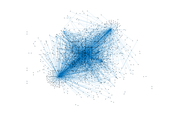### Degree distribution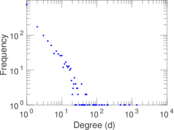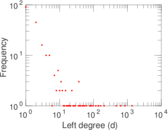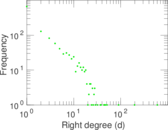### Cumulative degree distribution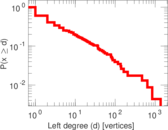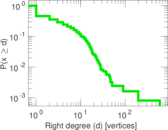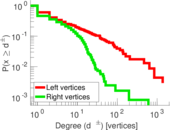### Lorenz curve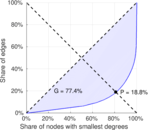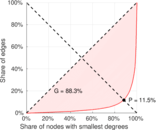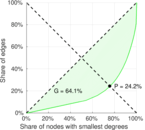### Spectral distribution of the adjacency matrix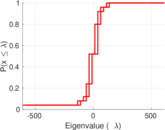### Spectral distribution of the normalized adjacency matrix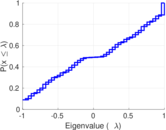### Spectral distribution of the Laplacian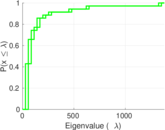### Spectral graph drawing based on the adjacency matrix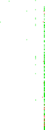### Spectral graph drawing based on the Laplacian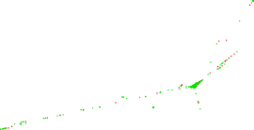### Spectral graph drawing based on the normalized adjacency matrix### Degree assortativity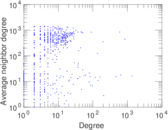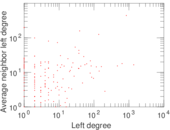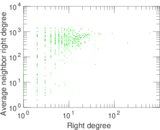### Zipf plot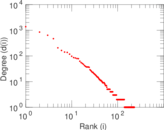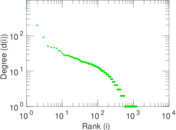### Hop distribution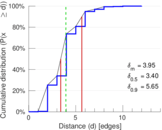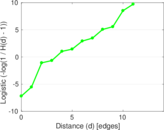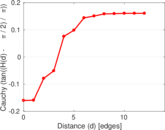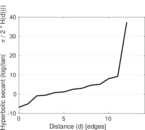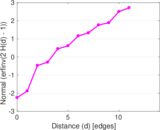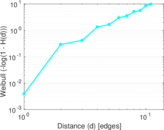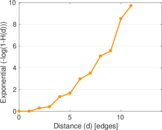### Double Laplacian graph drawing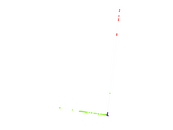### Delaunay graph drawing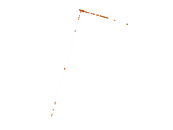### Edge weight/multiplicity distribution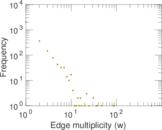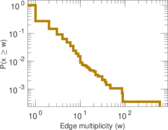### Temporal distribution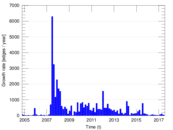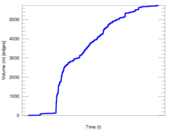### Temporal hop distribution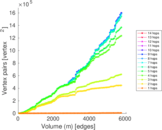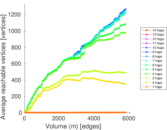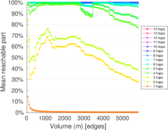### Diameter/density evolution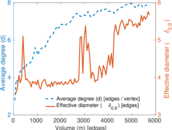### Matrix decompositions plots